## Blog

FUN ACTIVITIES
Hits
290
Date
04 / 22 / 2019

### Quiz) Find the sum of the numbers from 1 to 100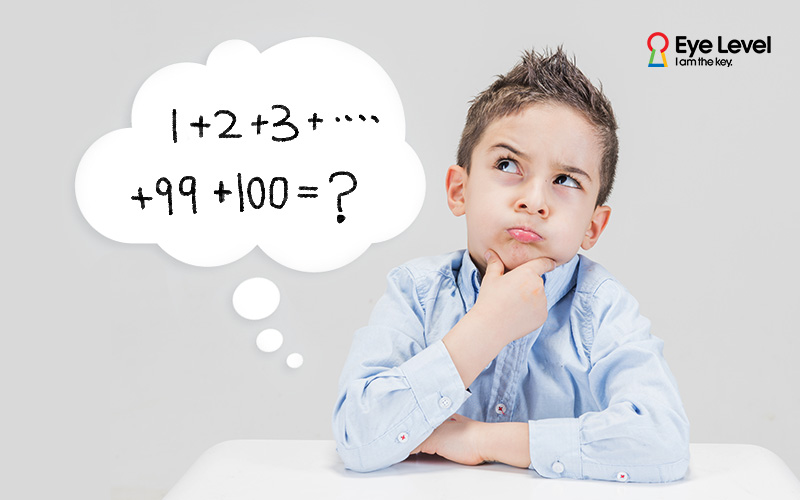Are you trying to find the answer by adding one by one starting from 1?

This is what is likely to happen when a student is not aware of problem solving strategies.

There are many ways to solve math problems. However, when students solve math problems in ineffective ways, it takes a lot of time and the answers are very often wrong.

Problem-solving strategies help students solve problems in easy and effective ways.

Let’s try to solve a similar problem!

✅Q. Find the sum of the numbers from 1 to 20. Answer the questions.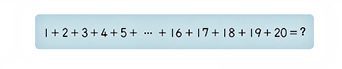This problem can be solved by the problem-solving strategy known as ‘Pattern Recognition’.

Step 1. Understand the problem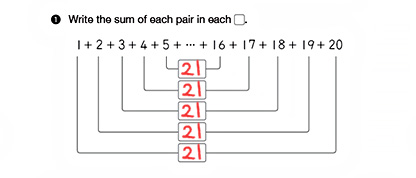Step 2. Discover patterns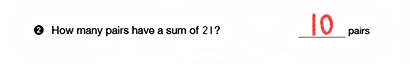Step 3. Find the formula and solve the question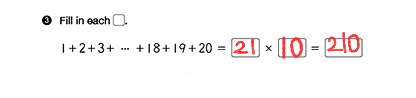Students can develop their problem-solving abilities by following the steps given above. Then, they will be able to solve the problem of finding the sum of the numbers even to the 1000th figure. These and similar types of problems can be solved by the same principle. Eye Level Math booklets are designed to enable students to practice and develop their problem-solving abilities on their own.

Ref. EYE LEVEL MATH CRITICAL THINKING MATH LV. 18​ (Patterns and Relationships by ‘Pattern Recognition’)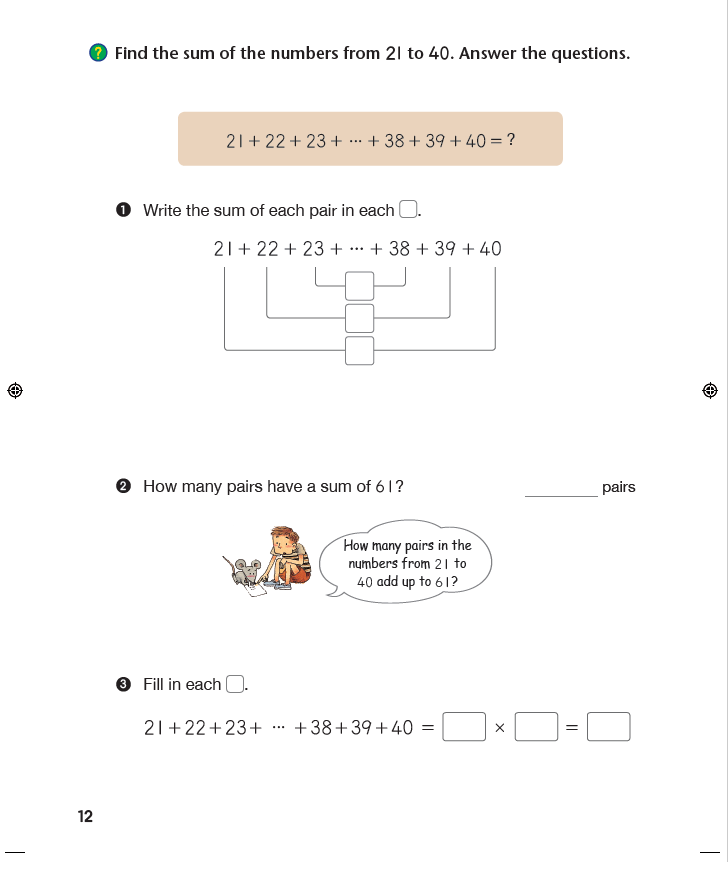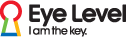▶ What is Eye Level Math Program?

​ Do you want to get a free diagnostic test? Contact us